# Problems of the Week

Contribute a problem

# 2017-10-16 Basic

If it's $\color{#3D99F6}\text{O}$'s turn, can $\color{#3D99F6}\text{O}$ force a win?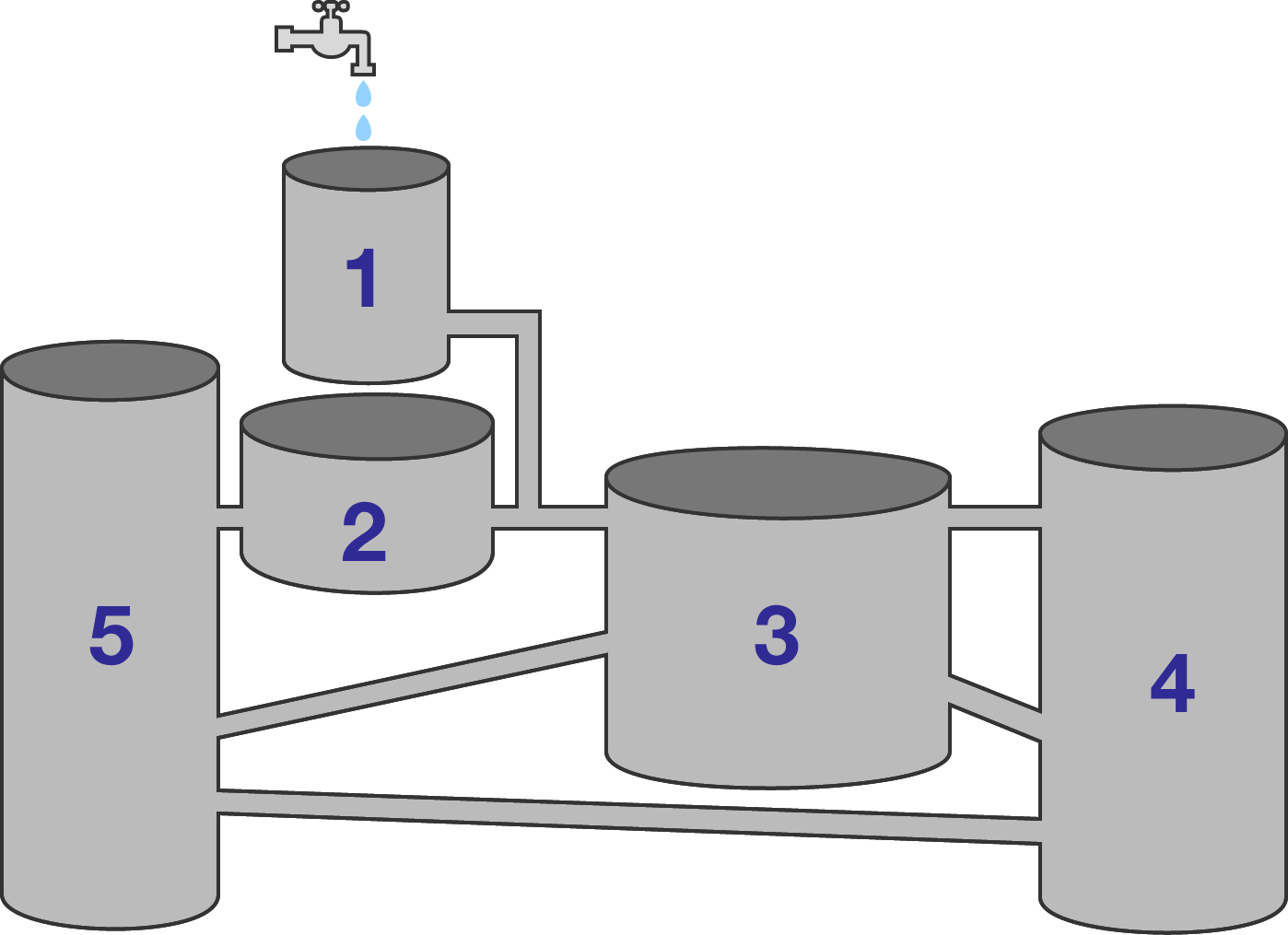In the diagram, which tank will become full first?


Details and Assumptions:

1. Water flows out of the faucet so slowly that the flow never exceeds the carrying capacity of the pipes.
2. All tanks are open at the top. All pipes have equal diameters.
3. The centers of the tops (and bottoms) of the tanks all lie in the same plane. So, tanks that are higher in the diagram are actually higher.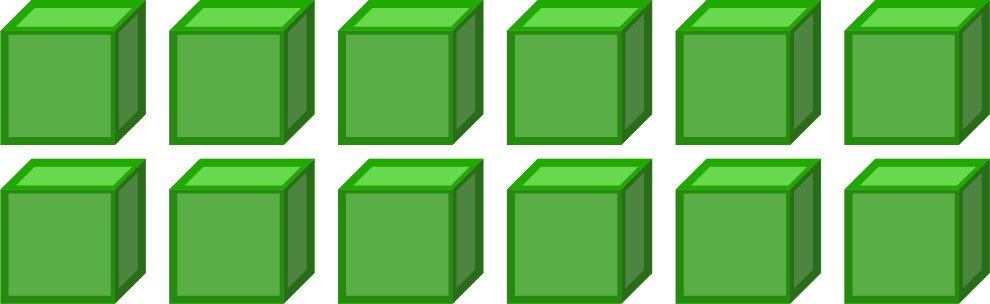I want to arrange 12 unit cubes into a rectangular cuboid so that its surface area is maximized.

One way I can do it is by arranging each of these cubes from left to right. Can I increase the surface area further?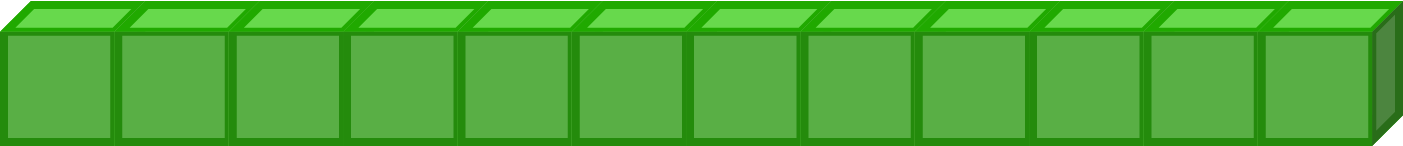Note: A rectangular cuboid is a three-dimensional solid with 6 rectangular faces.

A convex quadrilateral has 2 diagonals. A convex pentagon has 5 diagonals. A convex hexagon has 9 diagonals.

How many diagonals does a convex decagon (10 sides) have?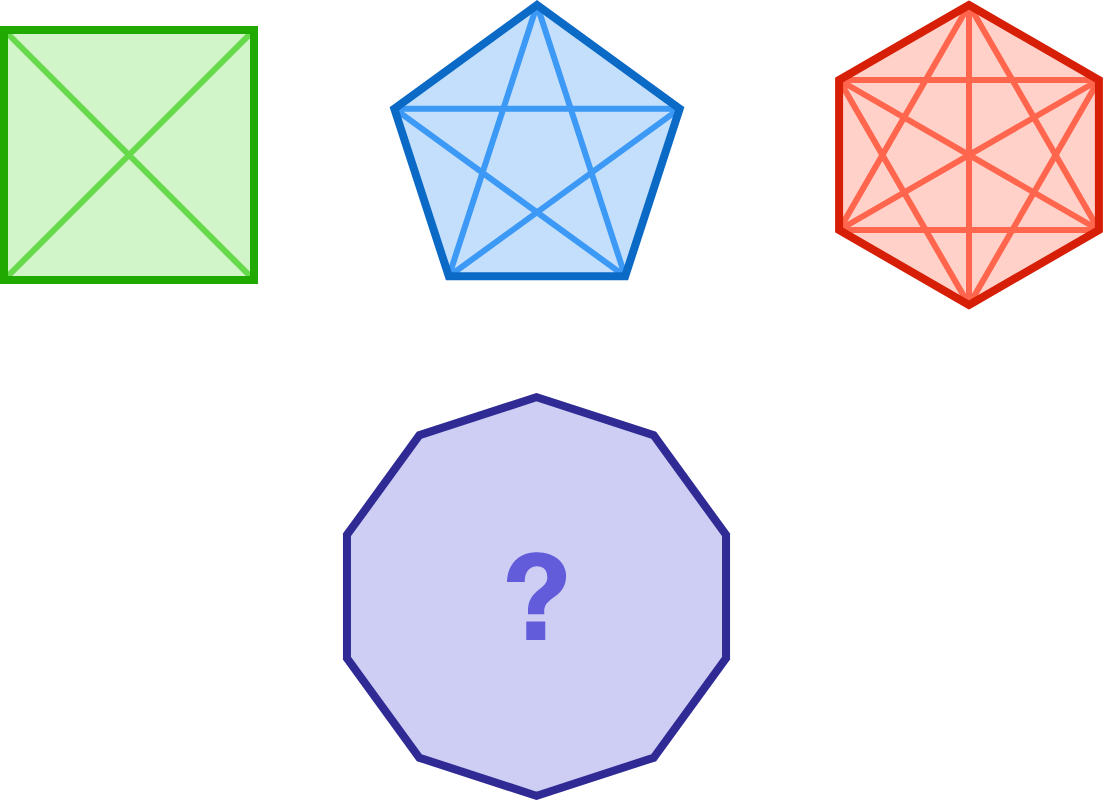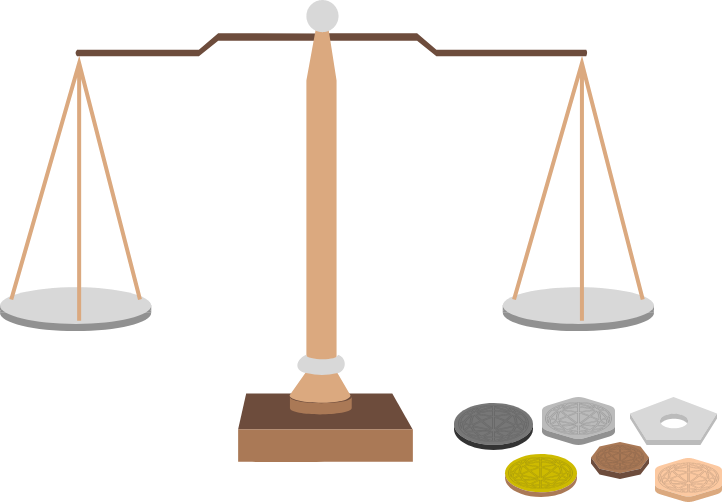Chris has 6 coins of distinct weights and a beam balance with two pans.

Can he find both the heaviest and the lightest coins by using the balance 7 times?

×